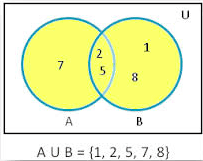# Python Set Union(): Complete Guide

The union() method returns the set that contains all items from the original set and all the elements from the specified sets. You can specify as many sets as you want, separated by the commas.

If the element is present in more than one set, the result will contain only one item’s appearance. If A = {2,5,7} and B = {1,2,5,8} are two sets, then the Union of these two sets will be AUB={1,2,5,7,8}, though 2 and 5 are common in both the sets, but in result set it will be taken only once.## Python set union()

The set union() is a built-in Python method that returns the set that contains all elements from the original set and all elements from the specified sets. You can specify as many sets you want, separated by the commas. If the item is present in more than one set, the result will contain only one appearance of this item.

### Syntax

`set1.union(set2,set3,set4...)`

This method takes arbitrary numbers of elements as parameters.

### Return Value

The set union() method returns a set that contains the union of all the given sets. If no parameter is passed as an argument, it returns a copy of the calling set, which means set1.

See the following code example.

```# app.py

# Declaring sets

# Even nums between 2 and 10
set1 = {2, 4, 6, 8, 10}
# Multiple of 3 between 1 to 10
set2 = {3, 6, 9}
# All prime numbers between 1 to 10
set3 = {2, 3, 5, 7}
# priting both the sets
print("Set1 is: ", set1)
print("Set2 is : ", set2)
print("Set3 is: ", set3)
# Now we will find Union of these sets
print("Union of set1 and set2 is: ", set1.union(set2))
print("Union of set1 and set2,set3 is: ", set1.union(set2, set3))
```

#### Output

```Set1 is:  {2, 4, 6, 8, 10}
Set2 is :  {9, 3, 6}
Set3 is:  {2, 3, 5, 7}
Union of set1 and set2 is:  {2, 3, 4, 6, 8, 9, 10}
Union of set1 and set2,set3 is:  {2, 3, 4, 5, 6, 7, 8, 9, 10}
```

## Python union using |

See the following code.

```# app.py

# Declaring sets

# Even nums between 2 and 10
set1 = {2, 4, 6, 8, 10}
# Multiple of 3 between 1 to 10
set2 = {3, 6, 9}
# All prime numbers between 1 to 10
set3 = {2, 3, 5, 7}
# priting both the sets
print("Set1 is: ", set1)
print("Set2 is : ", set2)
print("Set3 is: ", set3)
# Now we will find Union of these sets
print("Union of set1 and set2 is: ", set1 | set2)
print("Union of set1 and set2,set3 is: ", set1 | set2 | set3)
```

#### Output

```Set1 is:  {2, 4, 6, 8, 10}
Set2 is :  {9, 3, 6}
Set3 is:  {2, 3, 5, 7}
Union of set1 and set2 is:  {2, 3, 4, 6, 8, 9, 10}
Union of set1 and set2,set3 is:  {2, 3, 4, 5, 6, 7, 8, 9, 10}
```

Finally, Python Set Union() Method Example is over.

Python set symmetric_difference()

Python set remove()

Python set pop()

Python set issuperset()

Python set issubset()

Python set isdisjoint()

Python set intersection_update()

Python set intersection()